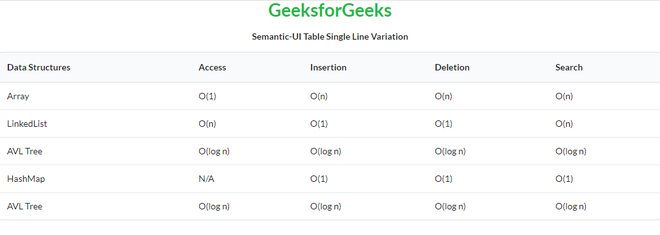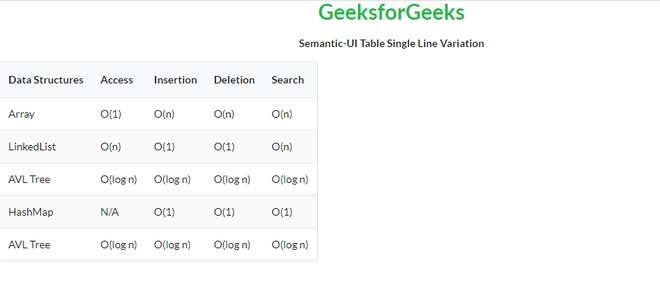GFG App
Open AppBrowser
Continue

# Semantic-UI Table Single Line Variation

Semantic UI is an open-source framework that uses CSS and jQuery to build great user interfaces. It is the same as a bootstrap for use and has great different elements to use to make your website look more amazing. It uses a class to add CSS to the elements.

Tables are an easy way to organize a lot of data. A table is an arrangement of data in rows and columns, or possibly in a more complex structure. Tables are widely used in communication, research, and data analysis. Tables are useful for various tasks such as presenting text information and numerical data. It can be used to compare two or more items in the tabular form layout. Tables are used to create databases. An HTML table and a Semantic UI table both are the same structurally.

In the case of Single Line Variation, we can specify that its cell contents should remain on a single line, and not wrap.

Semantic UI Table Single Line Variation Classes:

• single line: This class enables cell contents should remain on a single line, and not wrap.

Syntax:

```<table class="ui single line table">
<tr>
<td></td>
...
</tr>
...
</table>```

Example 1: This is a basic example illustrating Table Single Line Variation made using Semantic UI.

## HTML

 ` ` `<``html``> ` `    ``<``head``> ` `        ``<``title``>Semantic-UI Table Single Line Variation ` `        ``<``link` `href``=  ` `"https://cdnjs.cloudflare.com/ajax/libs/semantic-ui/2.4.1/semantic.min.css"`  `              ``rel``=``"stylesheet"``/> ` `        ``<``script` `src``= ` `"https://code.jquery.com/jquery-3.1.1.min.js"`  `                ``integrity``= ` `"sha256-hVVnYaiADRTO2PzUGmuLJr8BLUSjGIZsDYGmIJLv2b8="`  `                ``crossorigin``=``"anonymous"``> ` `        `` ` `        ``<``script` `src``= ` ` ``"https://cdnjs.cloudflare.com/ajax/libs/semantic-ui/2.4.1/semantic.min.js"``> ` `        `` ` `    `` ` `    ``<``body``> ` `        ``<``center``> ` `            ``<``h1` `class``=``"ui green header"``>GeeksforGeeks ` `            ``<``strong``>Semantic-UI Table Single Line Variation ` `        `` ` `        ``<``table` `class``=``"ui single line table"``> ` `          ``<``thead``> ` `              ``<``tr``> ` `                  ``<``th``>Data Structures ` `                  ``<``th``>Access ` `                  ``<``th``>Insertion ` `                  ``<``th``>Deletion ` `                  ``<``th``>Search ` `              `` ` `          `` ` `          ``<``tbody``> ` `            ``<``tr``> ` `              ``<``td``>Array ` `              ``<``td``>O(1) ` `              ``<``td``>O(n) ` `              ``<``td``>O(n) ` `              ``<``td``>O(n) ` `            `` ` `            ``<``tr``> ` `              ``<``td``>LinkedList ` `              ``<``td``>O(n) ` `              ``<``td``>O(1) ` `              ``<``td``>O(1) ` `              ``<``td``>O(n) ` `            `` ` `            ``<``tr``> ` `              ``<``td``>AVL Tree ` `              ``<``td``>O(log n) ` `              ``<``td``>O(log n) ` `              ``<``td``>O(log n) ` `              ``<``td``>O(log n) ` `            `` ` `            ``<``tr``> ` `              ``<``td``>HashMap ` `              ``<``td``>N/A ` `              ``<``td``>O(1) ` `              ``<``td``>O(1) ` `              ``<``td``>O(1) ` `            `` ` `            ``<``tr``> ` `              ``<``td``>AVL Tree ` `              ``<``td``>O(log n) ` `              ``<``td``>O(log n) ` `              ``<``td``>O(log n) ` `              ``<``td``>O(log n) ` `            `` ` `        `` ` `      `` ` `    `` ` ``

Output:Semantic-UI Table Single Line Variation

Example 2: This is a basic example illustrating Striped Collapsing Table Single Line Variation made using Semantic UI.

## HTML

 ` ` `<``html``> ` `    ``<``head``> ` `        ``<``title``>Semantic-UI Table Single Line Variation ` `        ``<``link` `href``=  ` `"https://cdnjs.cloudflare.com/ajax/libs/semantic-ui/2.4.1/semantic.min.css"` `              ``rel``=``"stylesheet"` `/> ` `        ``<``script` `src``= ` `"https://code.jquery.com/jquery-3.1.1.min.js"`  `                ``integrity``= ` `"sha256-hVVnYaiADRTO2PzUGmuLJr8BLUSjGIZsDYGmIJLv2b8="` `                ``crossorigin``=``"anonymous"``> ` `        ``<``script` `src``= ` ` ``"https://cdnjs.cloudflare.com/ajax/libs/semantic-ui/2.4.1/semantic.min.js"``> ` `        `` ` `    `` ` `    ``<``body``> ` `        ``<``center``> ` `            ``<``h1` `class``=``"ui green header"``>GeeksforGeeks ` `            ``<``strong``>Semantic-UI Table Single Line Variation ` `        `` ` `        ``<``table` `class``=``"ui single striped collapsing line table"``> ` `          ``<``thead``> ` `              ``<``tr``> ` `                  ``<``th``>Data Structures ` `                  ``<``th``>Access ` `                  ``<``th``>Insertion ` `                  ``<``th``>Deletion ` `                  ``<``th``>Search ` `              `` ` `          `` ` `          ``<``tbody``> ` `            ``<``tr``> ` `              ``<``td``>Array ` `              ``<``td``>O(1) ` `              ``<``td``>O(n) ` `              ``<``td``>O(n) ` `              ``<``td``>O(n) ` `            `` ` `            ``<``tr``> ` `              ``<``td``>LinkedList ` `              ``<``td``>O(n) ` `              ``<``td``>O(1) ` `              ``<``td``>O(1) ` `              ``<``td``>O(n) ` `            `` ` `            ``<``tr``> ` `              ``<``td``>AVL Tree ` `              ``<``td``>O(log n) ` `              ``<``td``>O(log n) ` `              ``<``td``>O(log n) ` `              ``<``td``>O(log n) ` `            `` ` `            ``<``tr``> ` `              ``<``td``>HashMap ` `              ``<``td``>N/A ` `              ``<``td``>O(1) ` `              ``<``td``>O(1) ` `              ``<``td``>O(1) ` `            `` ` `            ``<``tr``> ` `              ``<``td``>AVL Tree ` `              ``<``td``>O(log n) ` `              ``<``td``>O(log n) ` `              ``<``td``>O(log n) ` `              ``<``td``>O(log n) ` `            `` ` `        `` ` `      `` ` `    `` ` ``

Output:My Personal Notes arrow_drop_up